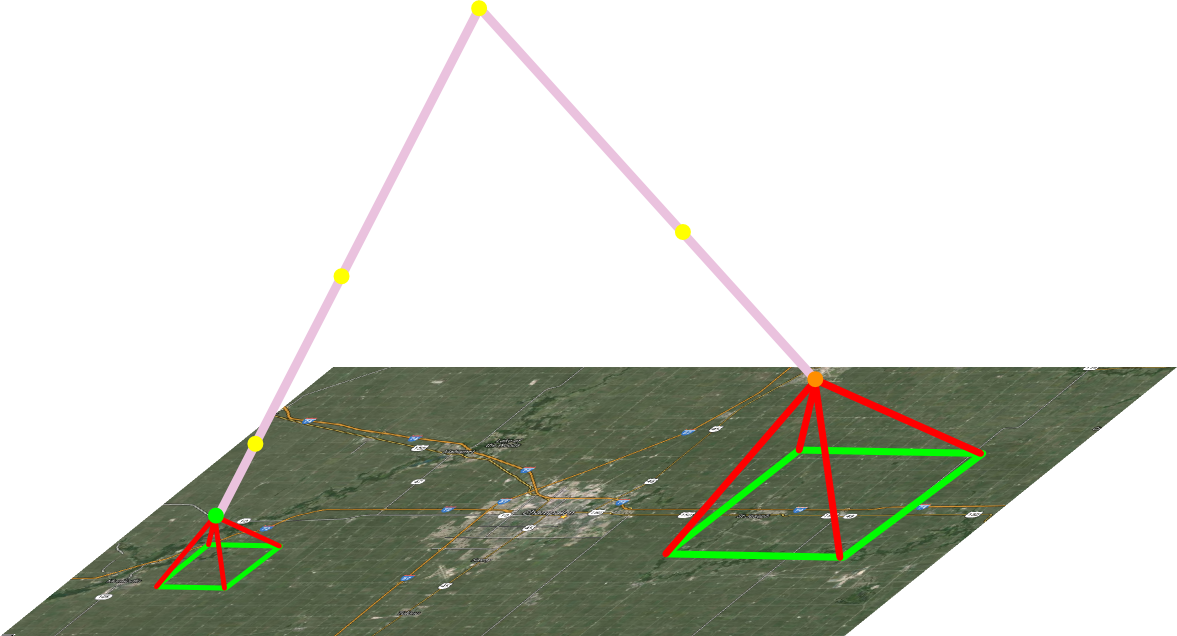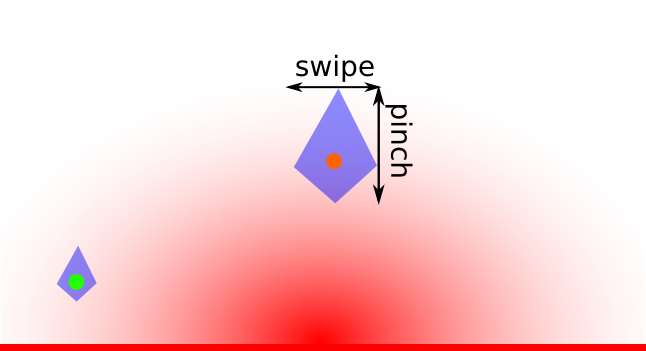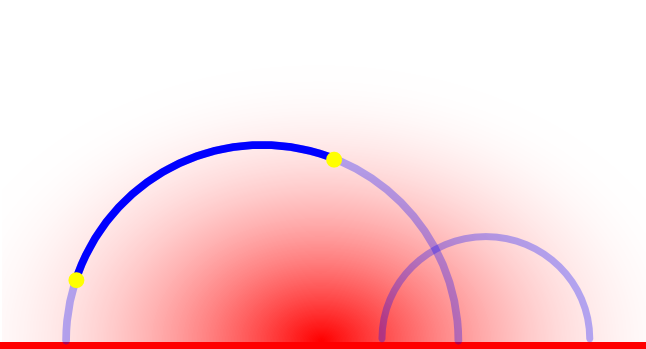$$\def\Real{\mathbb{R}} \def\views{\mathbb{G}} \def\earth{\mathbb{E}} \def\hsp{\mathbb{H}}$$

Google Maps and the Space of Views

Let’s consider the geometry hidden behind one of the best user interfaces in mobile apps ever, – the smartphone maps, the ones which you can swipe, flick and pinch. We are not talking here about the incredible engine that generates the maps on the fly as your finger waddles over the screen – just about the interface, which in a very intuitive and remarkably efficient way allows you to move between various maps.

So – it is the navigation of views in your smartphone map app we are concerned with, not navigating the Earth. In the process of navigating the smartphone maps – let’s call them Google maps, as Google API is the horse behind most of these apps – we are moving from one view to another.

What is a view?

Assume the Earth to be flat. (Not an unreasonable simplification: after all, this is how Google maps sees the Earth: remember the full zoom out? it is a periodically repeating flat map, an unfolding of a cylinder onto which the spherical Earth surface is projected, like Mercator or Miller projections.) We will denote this flat plane (of points on the Google maps) as $$\earth$$.

In any case, we are interested here not in the Earth or its map per se, but in what we actually see in the application. The collection of such views forms the space of views $$\views$$ that can be identified with
$\views=\earth\times\Real_+:$
here the point of $$\earth$$ is where a view is centered, and the second coordinate indicates the scale of the view – or, mnemonically, the height of one’s vantage point.

A couple of views are shown below as the apexes of the pyramids based on the areas visible on the screen:Navigating the Space of Views

The picture above also shows a way to navigate between the views – green and orange – in the app on your smartphone: it consists of five steps: three zoom outs, and two zoom ins, with recentering.

This is the natural way to navigate, and intuitively most efficient one.

Can we formalize this notion? What is the optimal way to navigate the space of views, say going from one screen showing downtown Urbana to another, with Oahu island?

To properly formulate the question we need to introduce some metric on the space of views. A natural candidate would be the finger distance, which we can roughly define as the minimal number of finger movements (swipes, pinches, what not) one needs to go from one view to another. The picture below show a schematic region around a couple of views achievable using one finger movement, like swipe or pinch.We are not going to specify too precisely the neuro-physiology of the finger movements. For our purposes, it will be enough to establish just a few properties of the finger distance $$d_\views$$.

Most importantly, this metric is invariant with respect to shifts along the Earth plane $$\earth$$ – the trouble of going from a point A to the point 100 miles northwest from it does not depend where the point A is, and is invariant with respect to rescaling – if it takes one pinch to double the height of your view, it does not matter what is this initial height.

In other words, the finger metric $$d_\views$$ is invariant with respect to the group of shifts and rescalings of the space of views $$\views$$. This is a three-dimensional group acting transitively on $$\views$$.

Enter the hyperbolic space

Recall that $$\earth\times\Real_+=\Real^2\times\Real_+$$ is also a model for the standard three-dimensional hyperbolic space $$\hsp$$ equipped with the standard metric $$d_\hsp$$ – this is a Riemannian metric, with the Riemannian metric tensor given by
$|dz|^2=\frac{dx^2+dy^2+dh^2}{h^2},$
where $$(x,y)$$ are the coordinates in the horizontal plane (longitude and latitude, more or less), and $$h$$ is the height of the view.

We won’t go into the details of the beautiful geometry of the hyperbolic space, emphasizing just two aspects:

• First, the hyperbolic metric is invariant with respect to the same group – generated by the horizontal shifts and rescalings – as the finger metric. This is immediate from the explicit formula for the metric tensor above.
• Secondly, the shortest path – the geodesic line – in this metric between two points in $$\hsp=\Real^2\times\Real_+$$ is a semicircle in the vertical (i.e. perpendicular to the horizontal plane $$\{h=0\}$$) plane passing through these points, having the center on the horizontal plane (or, equivalently, intersecting the horizontal plane at straight angle).The figure above shows a couple of geodesics, in particular one passing through a pair of points.

Comparing $$d_\views$$ and $$d_\hsp$$.

The fact that both finger and hyperbolic metrics on the upper halfspace are invariant with respect to the same transitive group of symmetries immediately implies that

The space of views $$(\views, d_\views)$$ is quasi-isometric to the standard hyperbolic 3-space $$\hsp^3$$.

Recall that two metric structures (in this case, on the same topological space $$\views$$) are quasi-isometric if each of them is bounded from above by an affine-linear function of the other:
$d_\views\leq a_1+c_1d_\hsp; d_\hsp\leq a_2+c_2d_\views.$

This property in our case follows immediately from the fact that, obviously, a unit ball in $$d_\views$$ metric (the set of views attainable from a given one by one finger movement) is contained within a metric ball of some radius in (d_\hsp\), and vice versa. The joint invariant of both metrics with respect to the same transitive group implies that this is true for all points, and a simple reasoning (subdividing paths realizing the distances into the steps of length about 1) proves the claim.

Why this quasi-isometry is important in our situation? Primarily, because of the remarkable property that for spaces of non-positive curvature (like $$\hsp$$), the short paths of a quasi-isometric metric stay close to the geodesics of the original one: this result is called Morse lemma. So,

the geodesics of the hyperbolic space stay within the bounded distance from the geodesics of the finger metric.

In other words, the intuitive navigation algorithm:

	NAVIGATE(VIEW_START, VIEW_GOAL)
view=VIEW_START;
while VIEW_GOAL not in VIEW
zoom out;
end while;
center(VIEW_GOAL);
until VIEW==VIEW_GOAL;
zoom in;
end until;


is (up to a multiplicative constant) not only optimal – a quasi-geodesic in the finger metric – but is forced on the users by the geometry of the viewspace.

Some conclusions

Perhaps not too surprising, the hyperbolic geometry in the space of map views defined by the finger metric is (up to a constant) the best possible if one tries to minimize the distance between (most) of the view pairs of some reasonable compact subset of viewspace (for example, all views above some scale: Google maps allows the scales over 5 orders of magnitude or so, blanking at too fine a resolution).

Steps done by single motion connect finitely many views: being restricted by agility, image resolution etc. Thus the total number of steps needed is
at least $$\Omega(\log{V})$$, where $$V$$ is the number of views available at the given resolution level.